### Numerical Differentiation: Derivatives Using Interpolation Functions

### Derivatives Using Interpolation Functions

The basic and high-accuracy formulas derived above were based on equally spaced data, i.e., the distance between the points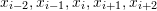is constant. It is possible to derive formulas for unequally spaced data such that the derivatives would be a function of the spacings close to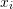. Another method is to fit a piecewise interpolation function of a particular order to the data and then calculate its derivative as described previously. This technique in fact provides better estimates for higher values of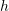as shown in the following tools. However, the disadvantage is that depending on the order of the interpolation function, higher derivatives are not available.

#### First Order Interpolation

The first order interpolation provides results for the first derivatives that are exactly similar to the results using forward finite difference. However, a first order interpolation function predicts zero second and higher derivatives. In the following tool,defines the interval at which the Runge function is sampled. The first derivative is calculated similar to the forward finite difference method. The higher derivatives are zero. The tool overlays the actual derivative with that predicted using the interpolation function. The black dots provide the values of the derivatives at the sampled points.

#### Second Order Interpolation

The second order interpolation provides much better results for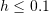compared with the finite difference formulas. However, the third and fourth derivatives are equal to zero. Change the value ofto see its effect on the results.

#### Third Order Interpolation

The third order interpolation (cubic splines) provides much better results for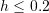compared with the finite difference formulas. However, the fourth derivative is equal to zero. Change the value ofto see its effect on the results.

The following Mathematica code can be used to generate the tools above.

View Mathematica Code
h = 0.2;
n = 2/h + 1;
y = 1/(1 + 25 x^2);
yp = D[y, x];
ypp = D[yp, x];
yppp = D[ypp, x];
ypppp = D[yppp, x];
Data = Table[{-1 + h*(i - 1), (y /. x -> -1 + h*(i - 1))}, {i, 1, n}];
y2 = Interpolation[Data, Method -> "Spline", InterpolationOrder -> 1];
ypInter = D[y2[x], x];
yppInter = D[y2[x], {x, 2}];
ypppInter = D[y2[x], {x, 3}];
yppppInter = D[y2[x], {x, 4}];
Data1 = Table[{Data[[i, 1]], (ypInter /. x -> Data[[i, 1]])}, {i, 1,     n}];
Data2 = Table[{Data[[i, 1]], (yppInter /. x -> Data[[i, 1]])}, {i, 1,    n}];
Data3 = Table[{Data[[i, 1]], (ypppInter /. x -> Data[[i, 1]])}, {i, 1,    n}];
Data4 = Table[{Data[[i, 1]], (yppppInter /. x -> Data[[i, 1]])}, {i,    1, n}];
a0 = Plot[{y, y2[x]}, {x, -1, 1},   Epilog -> {PointSize[Large], Point[Data]}, AxesLabel -> {"x", "y"},  BaseStyle -> Bold, ImageSize -> Medium, PlotRange -> All,  PlotLegends -> {"y actual", "y spline"},    PlotLabel -> "First order splines"];
a1 = Plot[{yp, ypInter}, {x, -1, 1},    Epilog -> {PointSize[Large], Point[Data1]},    AxesLabel -> {"x", "y'"}, BaseStyle -> Bold, ImageSize -> Medium,    PlotRange -> All, PlotLegends -> {"y'", "y'spline"},    PlotLabel -> "First derivative"];
a2 = Plot[{ypp, yppInter}, {x, -1, 1},    Epilog -> {PointSize[Large], Point[Data2]},    AxesLabel -> {"x", "y''"}, BaseStyle -> Bold, ImageSize -> Medium,    PlotRange -> All, PlotLegends -> {"y''", "y''spline"},    PlotLabel -> "Second derivative"];
a3 = Plot[{yppp, ypppInter}, {x, -1, 1},    Epilog -> {PointSize[Large], Point[Data3]},    AxesLabel -> {"x", "y''"}, BaseStyle -> Bold, ImageSize -> Medium,    PlotRange -> All, PlotLegends -> {"y'''", "y'''spline"},    PlotLabel -> "Third derivative"];
a4 = Plot[{ypppp, yppppInter}, {x, -1, 1},    Epilog -> {PointSize[Large], Point[Data4]},    AxesLabel -> {"x", "y''"}, BaseStyle -> Bold, ImageSize -> Medium,    PlotRange -> All, PlotLegends -> {"y''''", "y''''spline"},    PlotLabel -> "Fourth derivative"];
Grid[{{a0}, {a1}, {a2}, {a3}, {a4}}]

View Python Code
import numpy as np
import sympy as sp
import matplotlib.pyplot as plt
from scipy.interpolate import UnivariateSpline
# UnivariateSpline used here for its derivative function is similar to Interp1d

x = sp.symbols('x')
x_val = np.arange(-1, 1,0.01)
h = 0.2
n = int(2/h + 1)
y = 1/(1 + 25*x**2)
yp = y.diff(x, n=1)
ypp = yp.diff(x, n=2)
yppp = ypp.diff(x, n=3)
ypppp = yppp.diff(x, n=4)
Data = [[-1 + h*i, (y.subs(x, -1 + h*i))] for i in range(n)]

y2 = UnivariateSpline([point for point in Data],[point for point in Data], k=1,s=0)
ypInter = y2.derivative(1)
try: yppInter = y2.derivative(2)
except Exception as e: print(e); yppInter = lambda x: 0;
try: ypppInter = y2.derivative(3)
except Exception as e: print(e); ypppInter = lambda x: 0;
try: yppppInter = y2.derivative(4)
except Exception as e: print(e); yppppInter = lambda x: 0;

Data1 = [[point, ypInter(point)] for point in Data]
Data2 = [[point, yppInter(point)] for point in Data]
Data3 = [[point, ypppInter(point)] for point in Data]
Data4 = [[point, yppppInter(point)] for point in Data]

plt.title("First order splines")
plt.xlabel("x"); plt.ylabel("y")
plt.scatter([point for point in Data],[point for point in Data], c='k')
plt.plot(x_val, [y.subs(x,i) for i in x_val], label="y actual")
plt.plot(x_val, [y2(i) for i in x_val], label="y spline")
plt.legend(); plt.grid(); plt.show()

plt.title("First derivative")
plt.xlabel("x"); plt.ylabel("y'")
plt.scatter([point for point in Data1],[point for point in Data1], c='k')
plt.plot(x_val, [yp.subs(x,i) for i in x_val], label="y'")
plt.plot(x_val, [ypInter(i) for i in x_val], label="y'spline")
plt.legend(); plt.grid(); plt.show()

plt.title("Second derivative")
plt.xlabel("x"); plt.ylabel("y''")
plt.scatter([point for point in Data2],[point for point in Data2], c='k')
plt.plot(x_val, [ypp.subs(x,i) for i in x_val], label="y''")
plt.plot(x_val, [yppInter(i) for i in x_val], label="y''spline")
plt.legend(); plt.grid(); plt.show()

plt.title("Third derivative")
plt.xlabel("x"); plt.ylabel("y'''")
plt.scatter([point for point in Data3],[point for point in Data3], c='k')
plt.plot(x_val, [yppp.subs(x,i) for i in x_val], label="y'''")
plt.plot(x_val, [ypppInter(i) for i in x_val], label="y'''spline")
plt.legend(); plt.grid(); plt.show()

plt.title("Fourth derivative")
plt.xlabel("x"); plt.ylabel("y''''")
plt.scatter([point for point in Data4],[point for point in Data4], c='k')
plt.plot(x_val, [ypppp.subs(x,i) for i in x_val], label="y''''")
plt.plot(x_val, [yppppInter(i) for i in x_val], label="y''''spline")
plt.legend(); plt.grid(); plt.show()